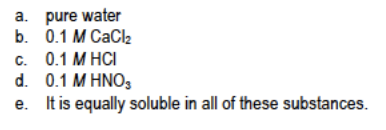# Problem: AgCl would be least soluble at 25°C in ______ .

###### FREE Expert Solution
83% (401 ratings)
###### FREE Expert Solution

We’re being asked to determine in which solvent AgCl would be least soluble in.

The common ion effect states that the solubility of a salt is lower in the presence of a common ion. This means if there is a higher concentration of a common ion present, the less soluble a compound will be. The dissociation of AgCl in water is as follows:

The chloride ion, Cl, has a charge of –1. Silver then has a charge of +1:

AgCl(s)  Ag+(aq) + Cl(aq)

Among the given choices, only choices b, CaCl2, and c, HCl, contain a common ion, Cl. Pure water and 0.10 M HNO3 don't contain common ions so the solubility of AgCl is not lowered in those.

We’ll now determine which between 0.10 M CaCl2 and 0.10 M HCl has a higher Cl concentration: a higher Cl (common ion) concentration will lead to lower solubility of AgCl.

83% (401 ratings)###### Problem Details

AgCl would be least soluble at 25°C in ______ .Frequently Asked Questions

What scientific concept do you need to know in order to solve this problem?

Our tutors have indicated that to solve this problem you will need to apply the Ksp concept. You can view video lessons to learn Ksp. Or if you need more Ksp practice, you can also practice Ksp practice problems.

What is the difficulty of this problem?

Our tutors rated the difficulty ofAgCl would be least soluble at 25°C in ______ . ...as low difficulty.

How long does this problem take to solve?

Our expert Chemistry tutor, Jules took 1 minute and 40 seconds to solve this problem. You can follow their steps in the video explanation above.

What professor is this problem relevant for?

Based on our data, we think this problem is relevant for Professor March's class at UAB.